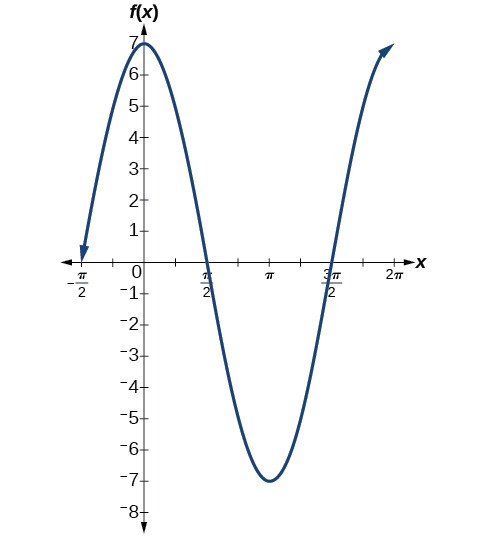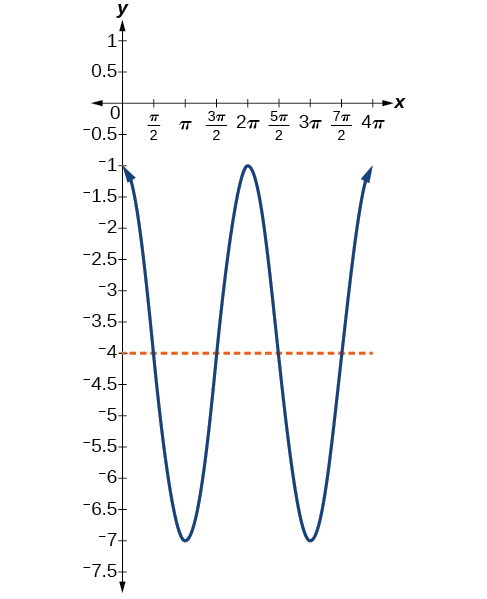# 6.1 Graphs of the sine and cosine functions  (Page 6/13)

 Page 6 / 13

## Identifying the properties of a sinusoidal function

Given $\text{\hspace{0.17em}}y=-2\mathrm{cos}\left(\frac{\pi }{2}x+\pi \right)+3,\text{\hspace{0.17em}}$ determine the amplitude, period, phase shift, and horizontal shift. Then graph the function.

Begin by comparing the equation to the general form and use the steps outlined in [link] .

$y=A\mathrm{cos}\left(Bx-C\right)+D$
• Step 1. The function is already written in general form.
• Step 2. Since $\text{\hspace{0.17em}}A=-2,\text{\hspace{0.17em}}$ the amplitude is $\text{\hspace{0.17em}}|A|=2.$
• Step 3. $\text{\hspace{0.17em}}|B|=\frac{\pi }{2},\text{\hspace{0.17em}}$ so the period is $\text{\hspace{0.17em}}P=\frac{2\pi }{|B|}=\frac{2\pi }{\frac{\pi }{2}}=2\pi \cdot \frac{2}{\pi }=4.\text{\hspace{0.17em}}$ The period is 4.
• Step 4. $\text{\hspace{0.17em}}C=-\pi ,$ so we calculate the phase shift as $\text{\hspace{0.17em}}\frac{C}{B}=\frac{-\pi ,}{\frac{\pi }{2}}=-\pi \cdot \frac{2}{\pi }=-2.\text{\hspace{0.17em}}$ The phase shift is $\text{\hspace{0.17em}}-2.$
• Step 5. $D=3,$ so the midline is $\text{\hspace{0.17em}}y=3,$ and the vertical shift is up 3.

Since $\text{\hspace{0.17em}}A\text{\hspace{0.17em}}$ is negative, the graph of the cosine function has been reflected about the x -axis.

[link] shows one cycle of the graph of the function.

## Using transformations of sine and cosine functions

We can use the transformations of sine and cosine functions in numerous applications. As mentioned at the beginning of the chapter, circular motion can be modeled using either the sine or cosine function    .

## Finding the vertical component of circular motion

A point rotates around a circle of radius 3 centered at the origin. Sketch a graph of the y -coordinate of the point as a function of the angle of rotation.

Recall that, for a point on a circle of radius r , the y -coordinate of the point is $\text{\hspace{0.17em}}y=r\text{\hspace{0.17em}}\mathrm{sin}\left(x\right),\text{\hspace{0.17em}}$ so in this case, we get the equation $\text{\hspace{0.17em}}y\left(x\right)=3\text{\hspace{0.17em}}\mathrm{sin}\left(x\right).\text{\hspace{0.17em}}$ The constant 3 causes a vertical stretch of the y -values of the function by a factor of 3, which we can see in the graph in [link] .

What is the amplitude of the function $\text{\hspace{0.17em}}f\left(x\right)=7\mathrm{cos}\left(x\right)?\text{\hspace{0.17em}}$ Sketch a graph of this function.

7## Finding the vertical component of circular motion

A circle with radius 3 ft is mounted with its center 4 ft off the ground. The point closest to the ground is labeled P , as shown in [link] . Sketch a graph of the height above the ground of the point $\text{\hspace{0.17em}}P\text{\hspace{0.17em}}$ as the circle is rotated; then find a function that gives the height in terms of the angle of rotation.

Sketching the height, we note that it will start 1 ft above the ground, then increase up to 7 ft above the ground, and continue to oscillate 3 ft above and below the center value of 4 ft, as shown in [link] .

Although we could use a transformation of either the sine or cosine function, we start by looking for characteristics that would make one function easier to use than the other. Let’s use a cosine function because it starts at the highest or lowest value, while a sine function    starts at the middle value. A standard cosine starts at the highest value, and this graph starts at the lowest value, so we need to incorporate a vertical reflection.

Second, we see that the graph oscillates 3 above and below the center, while a basic cosine has an amplitude of 1, so this graph has been vertically stretched by 3, as in the last example.

Finally, to move the center of the circle up to a height of 4, the graph has been vertically shifted up by 4. Putting these transformations together, we find that

$y=-3\mathrm{cos}\left(x\right)+4$

A weight is attached to a spring that is then hung from a board, as shown in [link] . As the spring oscillates up and down, the position $\text{\hspace{0.17em}}y\text{\hspace{0.17em}}$ of the weight relative to the board ranges from $\text{\hspace{0.17em}}–1\text{\hspace{0.17em}}$ in. (at time $\text{\hspace{0.17em}}x=0\right)\text{\hspace{0.17em}}$ to $\text{\hspace{0.17em}}–7\text{\hspace{0.17em}}$ in. (at time $\text{\hspace{0.17em}}x=\pi \right)\text{\hspace{0.17em}}$ below the board. Assume the position of $\text{\hspace{0.17em}}y\text{\hspace{0.17em}}$ is given as a sinusoidal function of $\text{\hspace{0.17em}}x.\text{\hspace{0.17em}}$ Sketch a graph of the function, and then find a cosine function that gives the position $\text{\hspace{0.17em}}y\text{\hspace{0.17em}}$ in terms of $\text{\hspace{0.17em}}x.$

$y=3\mathrm{cos}\left(x\right)-4$give me an example of a problem so that I can practice answering
x³+y³+z³=42
Robert
dont forget the cube in each variable ;)
Robert
of she solves that, well ... then she has a lot of computational force under her command ....
Walter
what is a function?
I want to learn about the law of exponent
explain this
what is functions?
A mathematical relation such that every input has only one out.
Spiro
yes..it is a relationo of orders pairs of sets one or more input that leads to a exactly one output.
Mubita
Is a rule that assigns to each element X in a set A exactly one element, called F(x), in a set B.
RichieRich
If the plane intersects the cone (either above or below) horizontally, what figure will be created?
can you not take the square root of a negative number
No because a negative times a negative is a positive. No matter what you do you can never multiply the same number by itself and end with a negative
lurverkitten
Actually you can. you get what's called an Imaginary number denoted by i which is represented on the complex plane. The reply above would be correct if we were still confined to the "real" number line.
Liam
Suppose P= {-3,1,3} Q={-3,-2-1} and R= {-2,2,3}.what is the intersection
can I get some pretty basic questions
In what way does set notation relate to function notation
Ama
is precalculus needed to take caculus
It depends on what you already know. Just test yourself with some precalculus questions. If you find them easy, you're good to go.
Spiro
the solution doesn't seem right for this problem
what is the domain of f(x)=x-4/x^2-2x-15 then
x is different from -5&3
Seid
All real x except 5 and - 3
Spiro
***youtu.be/ESxOXfh2Poc
Loree
how to prroved cos⁴x-sin⁴x= cos²x-sin²x are equal
Don't think that you can.
Elliott
By using some imaginary no.
Tanmay
how do you provided cos⁴x-sin⁴x = cos²x-sin²x are equal
What are the question marks for?
Elliott
Someone should please solve it for me Add 2over ×+3 +y-4 over 5 simplify (×+a)with square root of two -×root 2 all over a multiply 1over ×-y{(×-y)(×+y)} over ×y
For the first question, I got (3y-2)/15 Second one, I got Root 2 Third one, I got 1/(y to the fourth power) I dont if it's right cause I can barely understand the question.
Is under distribute property, inverse function, algebra and addition and multiplication function; so is a combined question
AbenaByByBy DanielrosenbergerBy Jesenia WoffordBy OpenStaxBy Gerr ZenBy OpenStaxBy OpenStaxBy Richley CrapoBy Anh DaoBy Kimberly NicholsBy OpenStax